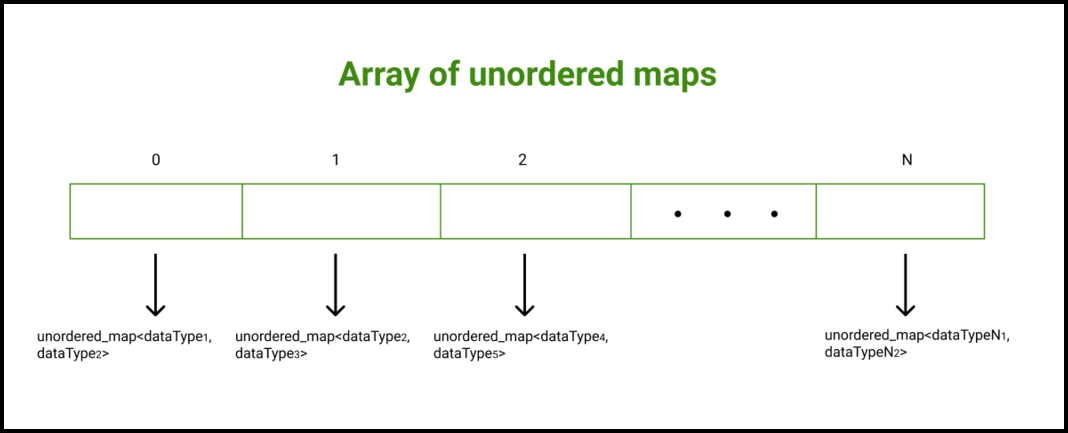GeeksforGeeks App
Open AppBrowser
Continue

# Array of unordered maps in C++ with Examples

What is an array?

An array in any programming language is a data structure that is used to store elements or data items of similar data types at contiguous memory locations and elements can be accessed randomly using indices of an array. Arrays are efficient when we want to store a large number of elements that too of similar data types.

What is Unordered Map?

Unordered_map is an associated container that stores elements formed by the combination of key-value and a mapped value. The key value is used to uniquely identify the element and the mapped value is the content associated with the key. Both key and value can be of any type predefined or user-defined. Elements in an unordered map are not arranged in any particular order.  Internally, an unordered map is implemented using a Hash Table.

Functions used with unordered map:

• at(): This function in C++ unordered_map returns the reference to the value with the element as key k.
• begin(): Returns an iterator pointing to the first element in the container in the unordered_map container
• end(): Returns an iterator pointing to the position past the last element in the container in the unordered_map container

This article focuses on how the array of unordered maps can be used in C++. An array of unordered maps can be quite useful while designing complex data structures.

Array of unordered maps

C++ allows us a facility to create an array of unordered maps. An array of unordered maps is an array in which each element is a map on its own.

Syntax:

unordered_map<<dataType1, dataType2>> myContainer[N];

Here,

N: The size of the array of unordered maps
dataType1: The dataType for the key
dataType2: The dataType for the valueExample 1: Below is the C++ program to implement the approach:

## C++

 `// C++ program to demonstrate the``// working of array of unordered maps in C++``#include ``using` `namespace` `std;`` ` `// Function to print unordered map elements``// specified at the index, "index"``void` `print(unordered_map<``int``, ``bool``>& myMap,``           ``int` `index)``{``    ``cout << ``"The unordered map elements stored "` `<< ``            ``"at the index "` `<< index << ``": \n"``;``    ``cout << ``"Key      Value\n"``;`` ` `    ``// Each element of the map is a pair on ``    ``// its own``    ``for` `(``auto` `pr : myMap) ``    ``{``        ``// Each element of the map is a pair ``        ``// on its own``        ``cout << pr.first << ``"         "` `<< ``                ``pr.second << ``'\n'``;``    ``}``    ``cout << ``'\n'``;``}`` ` `// Function to iterate over all the array``void` `print(unordered_map<``int``, ``           ``bool``>* myContainer, ``int` `n)``{``    ``// Iterating over myContainer elements``    ``// Each element is a map on its own``    ``for` `(``int` `i = 0; i < n; i++) ``    ``{``        ``print(myContainer[i], i);``    ``}``}`` ` `// Driver code``int` `main()``{``    ``// Declaring an array of unordered maps``    ``// In unordered map Key is of type int``    ``// Value is of type bool``    ``unordered_map<``int``, ``bool``> myContainer;`` ` `    ``// Mapping values to the unordered map ``    ``// stored at the index 0``    ``myContainer = ``true``;``    ``myContainer = ``false``;``    ``myContainer = ``true``;``    ``myContainer = ``false``;`` ` `    ``// Mapping values to the unordered map ``    ``// stored at the index 1``    ``myContainer = ``true``;``    ``myContainer = ``false``;``    ``myContainer = ``true``;``    ``myContainer = ``false``;`` ` `    ``// Mapping values to the unordered map ``    ``// stored at the index 2``    ``myContainer = ``true``;``    ``myContainer = ``false``;``    ``myContainer = ``true``;``    ``myContainer = ``false``;`` ` `    ``// Calling print function to iterate``    ``// over myContainer elements``    ``print(myContainer, 3);`` ` `    ``return` `0;``}`

Output

The unordered map elements stored at the index 0:
Key Value
25 0
20 1
10 1
15 0

The unordered map elements stored at the index 1:
Key Value
45 0
40 1
30 1
35 0

The unordered map elements stored at the index 2:
Key Value
65 0
60 1
50 1
55 0

Example 2: Below is the C++ program to implement the approach:

## C++

 `// C++ program to demonstrate the``// working of array of maps in C++``#include ``using` `namespace` `std;`` ` `// Function to print unordered map elements ``// specified at the index, "index"``void` `print(unordered_map& myMap, ``int` `index)``{``    ``cout << ``"The unordered map elements stored "` `<< ``            ``"at the index "` `<< index << ``": \n"``;``    ``cout << ``"Key      Value\n"``;`` ` `    ``// Each element of the unordered map is ``    ``// a pair on its own``    ``for` `(``auto` `pr : myMap) ``    ``{``        ``cout << pr.first << ``"      "` `<< ``                ``pr.second << ``'\n'``;``    ``}``    ``cout << ``'\n'``;``}`` ` `// Function to iterate over the unordered ``// map corresponding to an index``void` `print(unordered_map* myContainer, ``int` `n)``{``    ``for` `(``int` `i = 0; i < n; i++) ``    ``{``        ``print(myContainer[i], i);``    ``}``}`` ` `// Driver code``int` `main()``{``    ``// Declaring an array of unordered maps``    ``// In unordered map Key is of type string``    ``// Value is of type bool``    ``unordered_map myContainer;`` ` `    ``// Mapping values to the unordered map ``    ``// stored at the index 0``    ``myContainer[``"Code"``] = ``true``;``    ``myContainer[``"HTML"``] = ``false``;``    ``myContainer[``"Java"``] = ``true``;``    ``myContainer[``"Solo"``] = ``false``;`` ` `    ``// Mapping values to the unordered map ``    ``// stored at the index 1``    ``myContainer[``"PHP"``] = ``true``;``    ``myContainer[``"CSS"``] = ``false``;``    ``myContainer[``"C++"``] = ``true``;``    ``myContainer[``"Lab"``] = ``false``;`` ` `    ``// Mapping values to the unordered map ``    ``// stored at the index 2``    ``myContainer[``"Swift"``] = ``true``;``    ``myContainer[``"Cobol"``] = ``false``;``    ``myContainer[``"Fizzy"``] = ``true``;``    ``myContainer[``"Pizza"``] = ``false``;`` ` `    ``// Calling print function to print ``    ``// myContainer elements``    ``print(myContainer, 3);`` ` `    ``return` `0;``}`

Output

The unordered map elements stored at the index 0:
Key Value
Solo 0
Java 1
Code 1
HTML 0

The unordered map elements stored at the index 1:
Key Value
Lab 0
C++ 1
PHP 1
CSS 0

The unordered map elements stored at the index 2:
Key Value
Fizzy 1
Pizza 0
Swift 1
Cobol 0

My Personal Notes arrow_drop_up